Describing motion along a line

Start this free course now. Just create an account and sign in. Enrol and complete the course for a free statement of participation or digital badge if available.

Free course

# 4.5 The signed area under a general velocity-time graph

We have already seen (in Section 3.6) that in the context of uniform motion, the signed area under a particle's velocity-time graph, between two given times, represents the change in the particle's position during that time interval, with a positive area corresponding to displacement in the positive direction. In the case of uniform motion, the velocity-time graph was a horizontal line and the area under the graph was rectangular.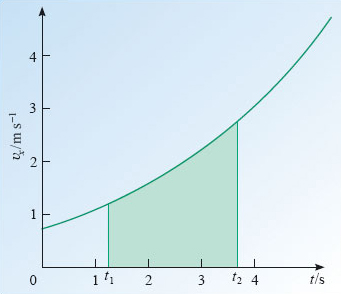Figure 33: The area under the velocity-time graph between t﻿1 and t﻿2 for an accelerating particle

Now, in the context of non-uniform motion, it seems natural to ask if the same interpretation can be given to the area under a general velocity-time graph, such as that between t1 and t2 in Figure 33. The answer to this is a definite yes, though a rigorous proof is beyond the scope of this course, so what follows is simply a plausibility argument.

In Figure 33, the colour-shaded area under the graph does not take the shape of a rectangle. However, it may be approximately represented by a sum of rectangular areas, as indicated in Figure 34. To produce Figure 34 we have broken the time between t1 and t2 into a number of small intervals, each of identical duration Δt and within each small interval, the velocity has been approximated by a constant, which can be taken to be the average velocity during that interval. As a result, the area of each rectangular strip in Figure 34 represents the approximate change of position over a short time Δt and the sum of those areas represents the approximate change of position over the interval t1 to t2. Now, if we were to repeat this process while using a smaller value for Δt, as in Figure 35, then we would have more strips between t1 and t2; their total area, representing the approximate change in position between t1 and t2, would be an even closer approximation to the true area shown in Figure 33. Given these results, it seems reasonable to suppose that if we allowed Δt to become smaller and smaller, while the number of rectangular strips between t1 and t2 became correspondingly larger and larger, then we would eventually find that the area under the graph in Figure 33 was exactly equal to the change in position between t1 and t2.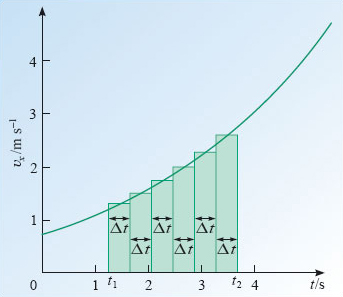Figure 34: The area under the velocity-time graph of Figure 33, broken up into thin rectangular strips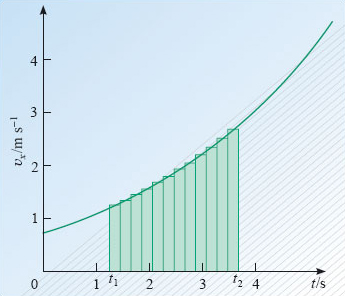Figure 35: The area under the velocity-time graph of Figure 33, broken up into even more rectangular strips by reducing the value of Δt

This conclusion is in fact correct and can be proved in a rigorous way by considering what mathematicians call a limit, in this case 'the limit as Δt tends to zero'. We shall not pursue that here, but we should note that it wasn't until the early nineteenth century that the mathematics of limits was properly formulated, although it was in use long before then. It is also worth pointing out that it was the development of the idea of a limit that finally laid Zeno's paradox to rest. Just as the increasing number of diminishing strips can have a finite total area, so the increasing number of smaller steps that Achilles must take to reach the tortoise can have a finite sum and be completed in a finite time. Rigorous mathematical reasoning agrees with our everyday experience in telling us that motion can exist and that athletes outrun tortoises!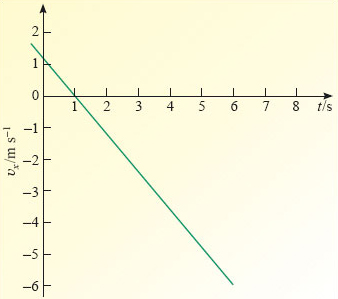Figure 36: The velocity-time graph for Self-assessment question 19

## Question 19

Figure 36 shows the velocity-time graph for a particle with constant acceleration.

(a) What is the displacement of the particle from its initial position after 6 s?

(b) What is the distance travelled by the particle between t = 2 s and t = 6 s﻿? (You may find it useful to know that the formula for the area of a triangle is: area = half the base × height, and that for the area of a trapezium is: area = base × (half the sum of the lengths of the parallel sides).)

(a) The displacement over the first 6 s is equal to the total signed area between the graph and the t-axis and between t = 0 s and t = 6 s in Figure 37. Recall that regions below the axis are regarded as negative areas. In this case the total area is composed of two triangles, one above the axis, one below. The total displacement is therefore given by

sx(6 s) = (1/2)×(1 s)×(1.2 m s−1) −(1/2)×(5 s)×(6 m s−1)

i.e. s﻿x﻿(﻿6 s﻿) = (﻿0.60 m﻿) −(15 m) = −14.4 m.

(b) The distance travelled between t = 2 s and t = 6 s will be the magnitude of the displacement over that time. Note that the displacement will be negative since it is represented by the area of the colour-shaded trapezium, which is entirely below the axis. However, the corresponding distance will be positive (since it is a magnitude) and will have the value

s = [(1/2) × (6 s − 2 s) × (6 m s−1 − 1.2 m s−1)] + [(6 s − 2 s) × 1.2 m s−1] = 14.4 m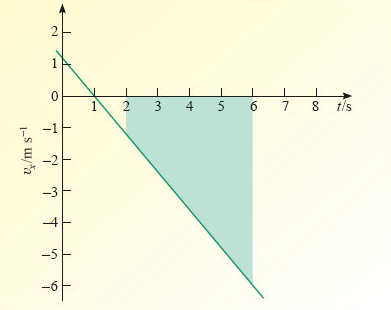Figure 37: The velocity-time graph for Self-assessment question 19
S207_2